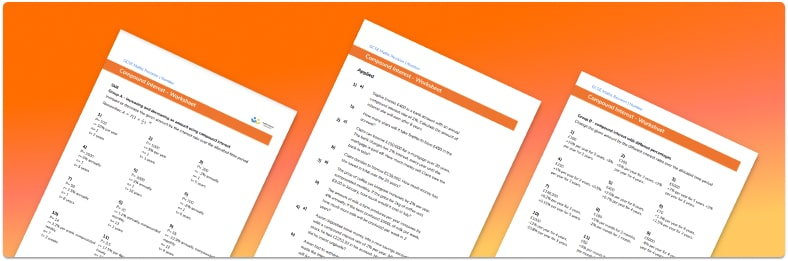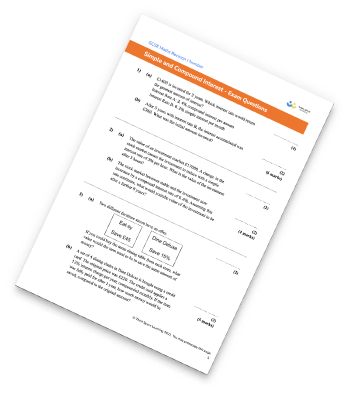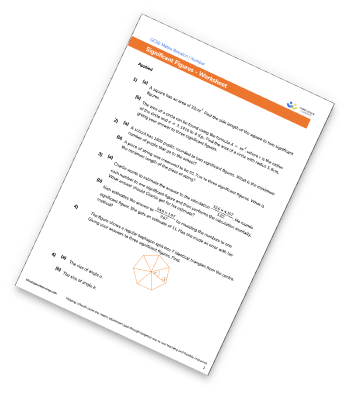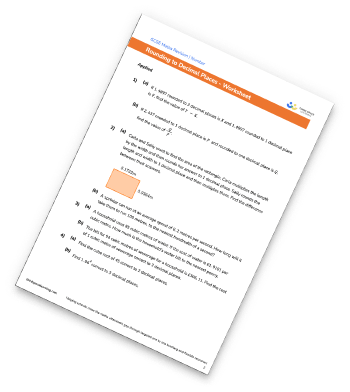# Compound Interest WorksheetHelp your students prepare for their Maths GCSE with this free compound interest worksheet of 44 questions and answers

• Section 1 of the compound interest worksheet contains 36 skills-based compound interest questions, in 3 groups to support differentiation
• Section 2 contains 4 applied compound interest questions with a mix of worded problems and deeper problem solving questions
• Section 3 contains 4 foundation and higher level GCSE exam style questions on compound interest
• Answers and a mark scheme for all compound interest questions are provided
• Questions follow variation theory with plenty of opportunities for students to work independently at their own level
• All questions created by fully qualified expert secondary maths teachers
• Suitable for GCSE maths revision for AQA, OCR and Edexcel exam boards
• To receive this resource and regular emails with more free resources, blog posts and other Third Space updates, enter your email address and click below.

• This field is for validation purposes and should be left unchanged.

You can unsubscribe at any time (each email we send will contain an easy way to unsubscribe). To find out more about how we use your data, see our privacy policy.

### Compound interest at a glance

Compound interest is interest that is calculated based on the original value of the investment as well as any interest previously earned.

For example, if £100 is invested in a savings account with a compound interest rate of 4% per annum then in the first year the account would earn £4 interest. At the beginning of the second year there would be £104 in the account and so the account would earn 4% of £104 and so on.

The annual compound interest formula is initial amount annual interest rate number of years. Annual interest rate is written as a percentage multiplier, so an interest rate of 4% would be a multiplier of 1.04. If interest is calculated over a different time period, such as semiannually, the ‘number of years’ would change to ‘number of times interest is paid’.

Compound depreciation works in the same way as compound interest, the only difference is that in this case, the percentage multiplier would be a decimal less than 1.

Looking forward, students can then progress to additional number worksheets, for example aor aFor more teaching and learning support on Number our GCSE maths lessons provide step by step support for all GCSE maths concepts.

## Related worksheets

Simple And Compound Interest WorksheetSignificant Figures WorksheetRounding To The Nearest 10, 100, And 1000 WorksheetRounding Decimals Worksheet## Do you have KS4 students who need more focused attention to succeed at GCSE?There will be students in your class who require individual attention to help them succeed in their maths GCSEs. In a class of 30, it’s not always easy to provide.

Help your students feel confident with exam-style questions and the strategies they’ll need to answer them correctly with our dedicated GCSE maths revision programme.

Lessons are selected to provide support where each student needs it most, and specially-trained GCSE maths tutors adapt the pitch and pace of each lesson. This ensures a personalised revision programme that raises grades and boosts confidence.

Find out more